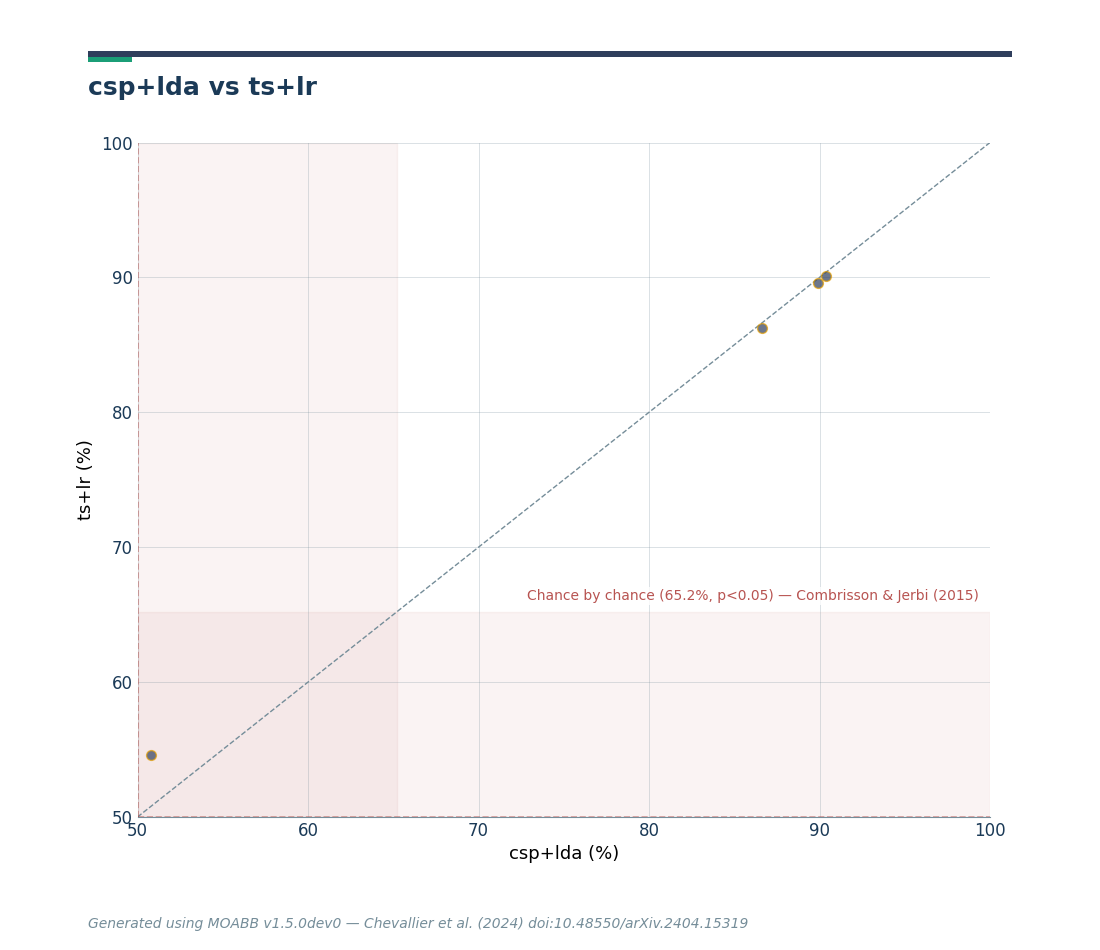Select electrodes and resampling¶

Within paradigm, it is possible to restrict analysis only to a subset of electrodes and to resample to a specific sampling rate. There is also a utility function to select common electrodes shared between datasets. This tutorial demonstrates how to use this functionality.

# Authors: Sylvain Chevallier <sylvain.chevallier@uvsq.fr>
#
import matplotlib.pyplot as plt
from mne.decoding import CSP
from pyriemann.estimation import Covariances
from pyriemann.tangentspace import TangentSpace
from sklearn.discriminant_analysis import LinearDiscriminantAnalysis as LDA
from sklearn.linear_model import LogisticRegression as LR
from sklearn.pipeline import make_pipeline

import moabb.analysis.plotting as moabb_plt
from moabb.datasets import BNCI2014001, Zhou2016
from moabb.datasets.utils import find_intersecting_channels
from moabb.evaluations import WithinSessionEvaluation

Datasets¶

Load 2 subjects of BNCI 2014-004 and Zhou2016 datasets, with 2 session each

subj = [1, 2]
datasets = [Zhou2016(), BNCI2014001()]
for d in datasets:
d.subject_list = subj

Restrict further analysis to specified channels, here C3, C4, and Cz. Also, use a specific resampling. In this example, all datasets are set to 200 Hz.

paradigm = LeftRightImagery(channels=["C3", "C4", "Cz"], resample=200.0)

Evaluation¶

The evaluation is conducted on with CSP+LDA, only on the 3 electrodes, with a sampling rate of 200 Hz.

csp_lda = make_pipeline(CSP(n_components=2), LDA())
ts_lr = make_pipeline(
Covariances(estimator="oas"), TangentSpace(metric="riemann"), LR(C=1.0)
)
results = evaluation.process({"csp+lda": csp_lda, "ts+lr": ts_lr})

Out:

score      time  samples  ... n_sessions    dataset  pipeline
0  0.878503  0.021208    144.0  ...          2   001-2014   csp+lda
1  0.869864  0.022608    144.0  ...          2   001-2014   csp+lda
2  0.483333  0.022285    144.0  ...          2   001-2014   csp+lda
3  0.518844  0.022681    144.0  ...          2   001-2014   csp+lda
4  0.865152  0.023656    119.0  ...          3  Zhou 2016   csp+lda

[5 rows x 9 columns]

Electrode selection¶

It is possible to select the electrodes that are shared by all datasets using the find_intersecting_channels function. Datasets that have 0 overlap with others are discarded. It returns the set of common channels, as well as the list of datasets with valid channels.

electrodes, datasets = find_intersecting_channels(datasets)
evaluation = WithinSessionEvaluation(
)
results = evaluation.process({"csp+lda": csp_lda, "ts+lr": ts_lr})

Out:

Searching dataset: Zhou2016
Searching dataset: BNCI2014001
score      time  samples  ... n_sessions    dataset  pipeline
0  0.857347  0.021013    144.0  ...          2   001-2014   csp+lda
1  0.856122  0.023419    144.0  ...          2   001-2014   csp+lda
2  0.442245  0.020887    144.0  ...          2   001-2014   csp+lda
3  0.531565  0.021407    144.0  ...          2   001-2014   csp+lda
4  0.850379  0.021628    119.0  ...          3  Zhou 2016   csp+lda

[5 rows x 9 columns]

Plot results¶

Compare the obtained results with the two pipelines, CSP+LDA and logistic regression computed in the tangent space of the covariance matrices.

fig = moabb_plt.paired_plot(results, "csp+lda", "ts+lr")
plt.show()Total running time of the script: ( 0 minutes 35.674 seconds)

Gallery generated by Sphinx-Gallery